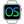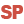HelpWLanguageWLanguage syntaxOperatorsAssignment operatorsSee alsoArithmetic operatorsAssignment operatorsBinary operatorsComparison operatorsExchange operator (<=>)Indirection operatorsList of operatorsLogical operatorsMultiline stringsOperator on AddressOperators on character stringsOther operatorsOverview
• Overview
• Simple assignment: =
• Multiple assignment
• Assignment by copy: <=
• Assignment by copy on the arrays
• Homonymic copy
• Assignment by taking reference: <-
• Remarks
WINDEVWEBDEVWINDEV MobileOthersAssignment operators
Overview
Several assignment operators are available:
Simple assignment: =
The simple assignment is used between all the simple types (integers, reals, strings, etc.) to copy the value of a variable into another one.
Its operating mode changes when it is used on complex types.

Multiple assignment

The '=' operator can also be used to assign several variables in a single line of code.
The following syntax is used:
( <Variable 1>, <Variable 2>, ... <Variable N> ) = ( <Value 1>, <Value 2>, ... <Value M> )
where:
• N represents the number of declared variables.
• M represents the number of values to assign.
Remarks:
• The expressions to the right of the '=' sign are evaluated from left to right.
• Then, the assignment operations of variables on the left of the sign are performed from left to right.
• There is no dependencies between the expressions on the right and the ones on the left. On the other hand, there can be a dependency between the variables on the left.
• If the number of variables is less than the number of values (N less than M), a compilation error will occur.
• If the number of variables is greater than the number of values (N greater than or equal to M), only the first variables will be assigned.
• To assign the same value to all the variables, all you have to do is use the following syntax:
( <Variable 1>, <Variable 2>, ... <Variable N> ) = <Value>

For example:
(x,y,z) = 0Not available.
Examples:
(x, y, z) = (5, 8, 12)
// x is set to 5, y is set to 8, z is set to 12

(x, y, z) = (25, 38)
// x is set to 25, y is set to 38, z is not assigned

(x, , z) = (14, 87)
// x is set to 14, y is not assigned, z is set to 87

(t[Subscript1()], t[Subscript2()]) = (Calc1(), calc2())
// The execution order of procedures is as follows:
// Calc1()
// Calc2()
// Subscript1()
// Subscript2()
// then t[Subscript1()] is assigned the value of Calc1()
// then t[Subscript2()] is assigned the value of Calc2()

(i, t[i]) = (1, "ABC")
// First assigns 1 to i
// Then assigns "ABC" in t to the subscript i
Assignment by copy: <=
The assignment by copy always forces the copy of the source element into the destination element.
A new memory area is allocated to store the copied value.

Assignment by copy on the arrays

The <= operator can be used on the arrays.
These arrays can have diferent types.
The assignment is using the implicit conversions of WLanguage (like the = operator would do =).
Example:
// Declare two arrays of same size and different types (integer and boolean)
arrIntegers is array of 3 by 2 int
arrBooleans is array of 3 by 2 booleans

// Fill the array of integers
arrIntegers[1,1] = -6
arrIntegers[1,2] = 9
arrIntegers[2,1] = 0

// Copy the array of integers into the array of booleans
arrBooleans <= arrIntegers

// Traces of array of booleans
// (Reminder: all the numbers other than zero are "True" in boolean logic)
Trace(arrBooleeans[1,1]) // Displays 1
Trace(arrBooleeans[1,2]) // Displays 1
Trace(arrBooleeans[2,1]) // Displays 0
Trace(arrBooleeans[2,2]) // Displays 0

Homonymic copy

The homonymic copy mechanism uses the <= operator to transfer the data from a structured element to another structured element.
During the copy, the members with the same name are automatically copied from the source structured element to the destination structured element. The type conversions will be automatically performed.
This allows you to:
• transfer the content of structure members to the items of an HFSQL file,
• transfer the members of advanced type (Email for example) to the members of a class.
The following structured types can be handled:
• structures,
• classes,
• HyperFileSQL files,
• queries,
• data sources.Limitation: the homonymic copy is not available for the Android applications.
Example: Copying classes
// Declare the classes
Class_1 is Class
common is int
member_1 is int
END

Class_2 is Class
common is int
member_2 is int
END

// Assignment
o1 is Class_1
o1.common = 1
o1.member_1 = 1

o2 is Class_2
o2.common = 2
o2.member_2 = 2

// Copy
o1 <= o2

// Result
// o1.common = 2
// o1.member_1 = 1
Remarks:
• The <= operator can be replaced with the = operator when the operating mode presents no ambiguity.
• For the structures and classes, each member can be followed by a "Mapping" attribute. This attribute is used to specify the name of the member that must be "mapped" to the structure member or to the class member.This extension attribute is not available.
Example:
// Declare the classes
Class_Simple_1 is Class
common is int
member_1 is int
END

Class_Mapping_1 is Class
nCommon is int, mapping = "common"
member_1 is int, mapping = ""
END

// Assignment
o1 is Class_Mapping_1
o1.nCommon = 1
o1.member_1 = 1

o2 is Class_Simple_1
o2.common = 2
o2.member_1 = 2

o1 <= o2

// Result
// o1.ncommon = 2
// o1.member_1 = 1
• For advanced types with dynamic sub-elements (for example, the xmlNode type or the structured elements of a web service), the sub-elements have priority over the standard properties of the type.
Example:
// Declare the classes
Class_xmlNode is Class
Name is string
Tag1 is string
Tag2 is string
END

// Assignment
o1 is Class_xmlNode
o1.Name = ""
o1.Tag1 = ""
o1.Tag2 = ""

c1 is xmlNode
c1..Name = "XML_node_with_Name_tag"
c1.Name= "Name"
c1.Tag1 = "Tag1"
c1.Tag2 = "Tag2"

o1 <= c1

// Result
// o1.Name = "Name"
// o1.Tag1 = "Tag1"
// o1.Tag2 = "Tag2"
• Special case: homonymic copy between structures or classes:
• The "mapping" attribute is allowed for the members of the structure or source or destination class (but not for both).
• If a derived member has the same name as a member of the base class or a mapping with the same name, only the name of the derived class is taken into account.
• Limit: The homonymic copy is not available:
• on .Net objects,
• on automation objects,
• if the source and the destination are advanced types with dynamic sub-elements.
Assignment by taking reference: <-
The assignment by taking reference forces the destination element to reference the same data area as the source element.
This assignment cannot be used with the simple types (integer, reals, strings, etc.).
This type of assignment can be used to directly handle:
Remarks
The operators for taking reference (=, <= et <-) return "Null" if the assignment or the take of reference is invalid. You have the ability to write the following code:
Object1 is BaseClass1 object
Object2 is DerivedClass object

Object1 <- Object2

IF Object1 = Null THEN
Trace("Object1 is not a DerivedClass or an ancestor class of DerivedClass.")
ELSE
Trace("Object1 is a DerivedClass or an ancestor class of DerivedClass.")
END
Minimum version required
• Version 15